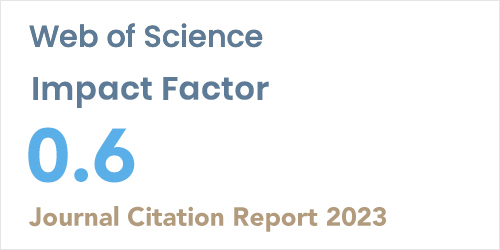ISSN: 1304-7191 | E-ISSN: 1304-7205
Volume: 41 Issue: 4 Year: 2023Linear Programming Problems with Fundamental Cut Matrices
1V.M.Glushkov Institute of Cybernetics, Kiev, UKRAINE
2Karabük University, Department of Computer Engineering, KARABUK
Sigma J Eng Nat Sci 2018; 36(3): 835-848

#### Abstract

In the paper, we consider a linear programming problem with con-straint matrices whose rows are 0, 1 characteristics vectors of fundamental cuts in a given undirected graph G = (V, E). We prove that the simplex algorithm finds an optimal solution in at most m-n+1 (m = |E|, n = |V|) iterations. We also consider the question whether a given binary matrix is a 0, 1 characteristic vector of fundamental cuts in the graph G.